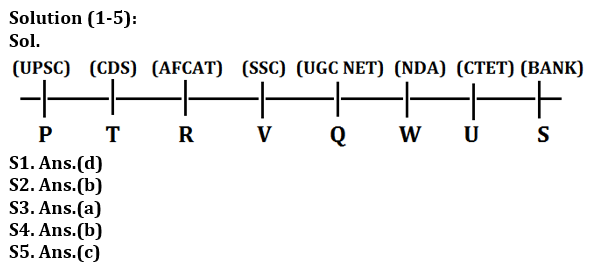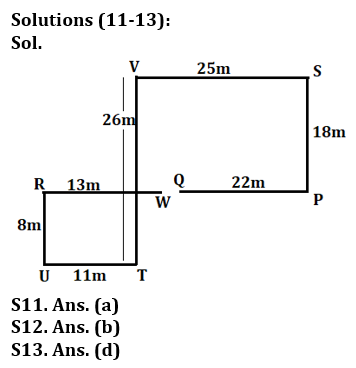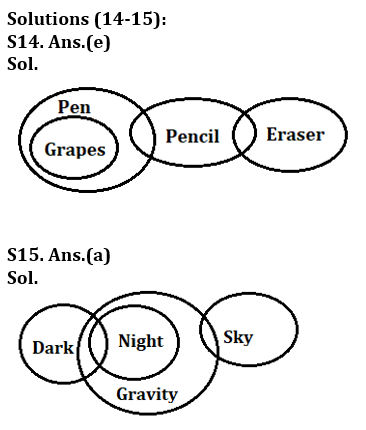Latest Banking jobs   »

# Reasoning Ability Quiz For Bank Foundation 2023-12th March

Directions (1-5): Study the following information carefully and answer the question given below.
Eight persons are sitting in a straight line and all are facing north direction. Each of them prepares for different competitive exam. S prepares for Bank and sits third to the right of Q. R sits immediate left of V who prepares for SSC. The one who prepares for AFCAT sits second to the left of Q. The one who prepares for UPSC sits third to the left of V. W prepares for NDA and he is an immediate neighbour of U who does not prepare for AFCAT. V is not an immediate neighbour of S. Persons who prepare for CDS and UPSC sit together. T sits third to the left of the one who prepares for UGC NET. W is not an immediate neighbour of S. P does not prepare for CTET.

Q1. Who among the following person prepare for CTET exam?
(a) T
(b) R
(c) W
(d) U
(e) None of these

Q2. Who among the following person sits third to the right V?
(a) the one who prepares for AFCAT
(b) The one who prepares for CTET
(c) S
(d) P
(e) None of these

Q3. What is the position of Q with respect to T?
(a) Third to the right
(b) Immediate Left
(c) Second to the right
(d) Immediate Right
(e) None of these

Q4. Which of the following statement is not true about U?
(a) U prepares for CTET
(b) U sits immediate right of S
(c) U does not prepare for UPSC
(d) Only two persons sit between U and the one who prepares for SSC
(e) Both (b) and (d)

Q5. Who among the following sits immediate right of the one who prepares for UGC NET?
(a) V
(b) U
(c) W
(d) P
(e) None of these

Direction (6-10): In these questions, relationships between elements is shown in the statements. These statements are followed by two conclusions. Give answer
(a) If only conclusion I follows.
(b) If only conclusion II follows.
(c) If either conclusion I or conclusion II follows.
(d) If neither conclusion I nor conclusion II follows.
(e) If both conclusions I and II follow.

Q6. Statements: G≤F=L≤J; J≤K=H
Conclusions: I. H=G II. G<H

Q7. Statements: P<R<S<T>U
Conclusions: I. U<R II. T>P

Q8. Statements: T>U≥V≥W; X<Y=W>Z
Conclusions: I. Z>U II. W<T

Q9. Statements: K<L<M<N; M<O<P
Conclusions: I. P>K II. N>O

Q10. Statements: B<A<C; A>D≤E
Conclusions: I. B≤E II. C>E

Direction (11-13): Study the given information and answer the following question.
Point P is 22m east of Q. V is 25m west of S. W is 13m east of R which is 8m north of U. T is 26m south of V. S is 18m north of P. T is 11m east of U.

Q11. What is the shortest distance between Q and W?
(a) 1m
(b) 5m
(c) 7m
(d) 2m
(e) None of these

Q12. Point W is in which direction with respect to V?
(a) South
(b) South-East
(c) East
(d) North-West
(e) South-West

Q13. Which of the following pair is in line?
(a) W, Q, S
(b) V, W, T
(c) R, U, T
(d) P, W, Q
(e) None of these

Directions (14-15): In each of the questions given below some statements are followed by some conclusions. You have to take the given statements to be true even if they seem to be at variance from commonly known facts. Read all the conclusions and then decide which of the given conclusions logically follows from the given statements disregarding commonly known facts.

Q14. Statements: All Grapes are Pen.
Some Pen are pencil.
Some Pencil are Eraser.
Conclusions: I. Some grapes are Pencil.
II. Some Pen are Eraser.
III. Some Grapes are Eraser.
(a) Only I follows
(b) Only II follows
(c) Only I and III follow
(d) Only III follows
(e) None follows

Q15. Statements: Some dark is night.
All night is gravity.
Some gravity is sky.
Conclusions: I. Some dark is gravity.
II. Some Sky are not dark.
III. All night are dark.
(a) Only I follows
(b) Only II and III follow
(c) Only I and III follow
(d) Only I and II follow
(e) None of these

SolutionsSolution (6-10):
S6. Ans.(c)
Sol.  I.H=G (False) II.G<H(False)
S7. Ans.(b)
Sol. I. U<R (False) II.T>P(True)
S8. Ans.(b)
Sol. I. Z>U (False) II.W<T(True)
S9. Ans.(a)
Sol. I. P>K (True) II. N>O(False)
S10. Ans.(d)
Sol. I. B≤E (False) II.C>E(False)## FAQs

### What is the selection process of the Bank Clerk?

The selection process of the Bank Clerk is Prelims & Mains.

#### Congratulations!Union Budget 2023-24: Free PDF